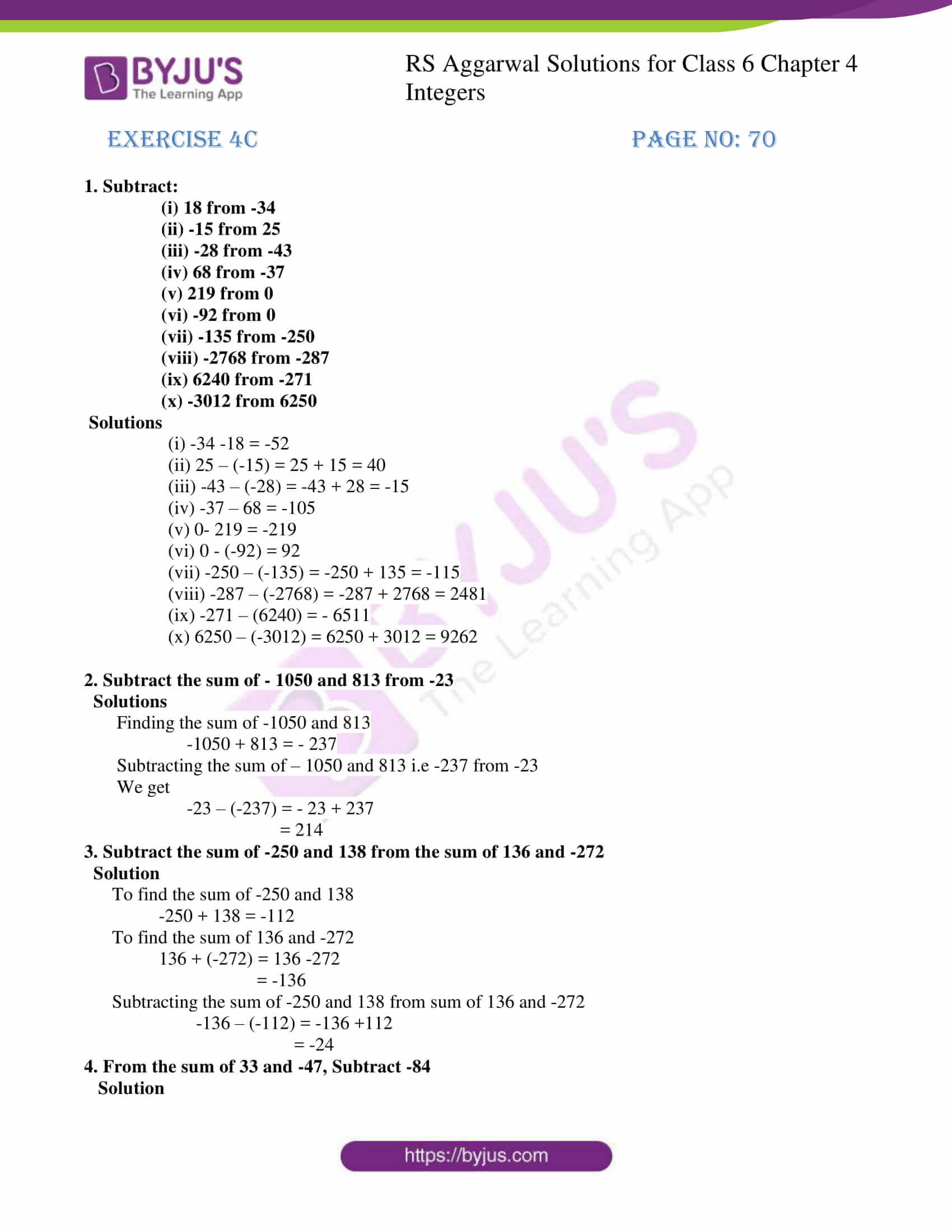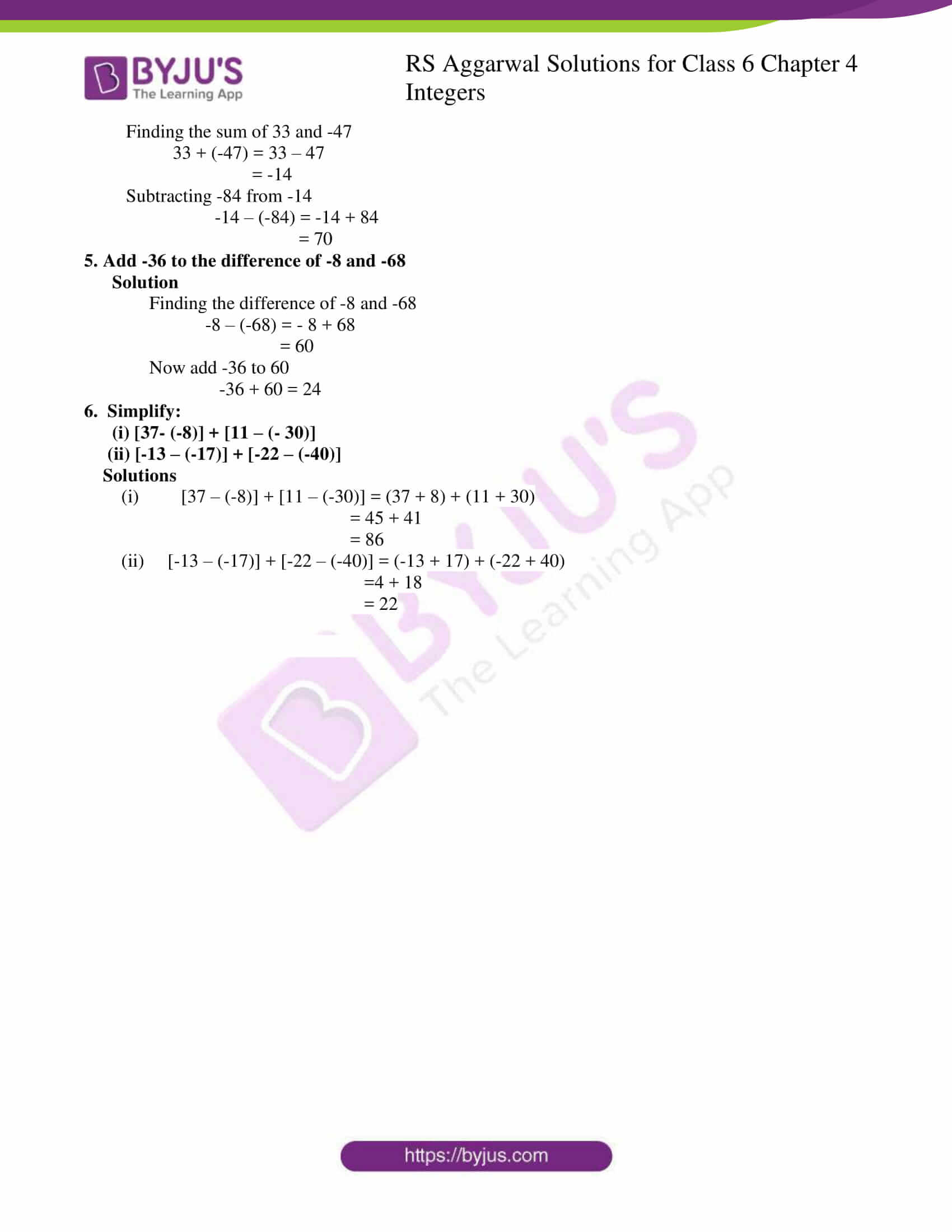# RS Aggarwal Solutions for Class 6 Chapter 4 Integers Exercise 4C

RS Aggarwal Solutions for Class 6, Chapter 4, Integers Exercise 4C are provided here in PDF format. Students can practice Exercise 4C, which helps in learning the subtraction of Integers effectively. These solutions are created by experts in Mathematics, thereby assisting students for a better understanding of the subject. Students who go through RS Aggarwal Solutions, score more marks in the examinations.

## Download PDF of RS Aggarwal Solutions for Class 6 Chapter 4 Integers Exercise 4C## Access answers to Maths RS Aggarwal Solutions for Class 6 Chapter 4 Integers Exercise 4C

1. Subtract:

(i) 18 from -34

(ii) -15 from 25

(iii) -28 from -43

(iv) 68 from -37

(v) 219 from 0

(vi) -92 from 0

(vii) -135 from -250

(viii) -2768 from -287

(ix) 6240 from -271

(x) -3012 from 6250

Solutions

(i) -34 -18 = -52

(ii) 25 – (-15) = 25 + 15 = 40

(iii) -43 – (-28) = -43 + 28 = -15

(iv) -37 – 68 = -105

(v) 0- 219 = -219

(vi) 0 – (-92) = 92

(vii) -250 – (-135) = -250 + 135 = -115

(viii) -287 – (-2768) = -287 + 2768 = 2481

(ix) -271 – (6240) = – 6511

(x) 6250 – (-3012) = 6250 + 3012 = 9262

2. Subtract the sum of – 1050 and 813 from -23

Solutions

Finding the sum of -1050 and 813

-1050 + 813 = – 237

Subtracting the sum of – 1050 and 813 i.e -237 from -23

We get

-23 – (-237) = – 23 + 237

= 214

3. Subtract the sum of -250 and 138 from the sum of 136 and -272

Solution

To find the sum of -250 and 138

-250 + 138 = -112

To find the sum of 136 and -272

136 + (-272) = 136 -272

= -136

Subtracting the sum of -250 and 138 from sum of 136 and -272

-136 – (-112) = -136 +112

= -24

4. From the sum of 33 and -47, Subtract -84

Solution

Finding the sum of 33 and -47

33 + (-47) = 33 – 47

= -14

Subtracting -84 from -14

-14 – (-84) = -14 + 84

= 70

5. Add -36 to the difference of -8 and -68

Solution

Finding the difference of -8 and -68

-8 – (-68) = – 8 + 68

= 60

-36 + 60 = 24

6. Simplify:

(i) [37- (-8)] + [11 – (- 30)]

(ii) [-13 – (-17)] + [-22 – (-40)]

Solutions

(i) [37 – (-8)] + [11 – (-30)] = (37 + 8) + (11 + 30)

= 45 + 41

= 86

(ii) [-13 – (-17)] + [-22 – (-40)] = (-13 + 17) + (-22 + 40)

=4 + 18

= 22